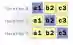# Scala For to, until, while Loop Examples

Use the for-loop on a List. Loop over a range of values with the for-to syntax.

For loops. A loop always looks to the future. It proceeds in order, 1 then 2 and 3. With functions we can apply logic to ranges all at once. But "for" is often simpler.Loop notes. With for, we can enumerate a collection like a list. We can use the "to" method to advance through a range of numbers. We must choose the best loop.

For example. Let us begin with a for-loop that enumerates a list. We create a list with 3 strings in it. Then we use the for-each loop on the list.
Tip: The syntax for a for-each loop in Scala is similar to that for Java. The language handles most of the iteration for us.
Val: We use the val keyword for the "ids" list, as we do not need to reassign it—we just loop over its contents.Scala program that uses for on string list object Program { def main(args: Array[String]): Unit = { // A list with 3 elements. val ids = List("a1", "b2", "c3") // Use a for-each loop over all the elements in the list. // ... Print the strings. for (id <- ids) println(id) } } Output a1 b2 c3

For to. Here we introduce a for-to loop. We use the to-keyword to specify to what number the loop advances. Once an iteration is past 5, the loop here terminates.
Scala program that uses for-to loop object Program { def main(args: Array[String]): Unit = { // Use a for-to loop to iterate over a range of numbers. // ... Start at 0 and continue until 5. for (i <- 0 to 5) println(i) } } Output 0 1 2 3 4 5

For until. With a for-loop we can also use the "until" keyword. To and until are methods that return an enumeration of numbers. Until() stops one number before the final number.
Scala program that uses for-until object Program { def main(args: Array[String]): Unit = { // With until, the final number is not included. // ... It is an exclusive bound. for (i <- 100 until 105) { println(i) } } } Output 100 101 102 103 104

For to, until syntax. Sometimes a program is clearer to read (or understand) with more exact syntax. Scala's syntax is flexible. We can omit, or add, parentheses in for-to and until loops.
Scala program that shows for-to, until syntax object Program { def main(args: Array[String]): Unit = { // Use parentheses around with to method. for (i <- 0.to(3)) { println("for to", i) } // Use parentheses with until. for (i <- 0.until(3)) { println("for until", i) } } } Output (for to,0) (for to,1) (for to,2) (for to,3) (for until,0) (for until,1) (for until,2)

Ranges. We can directly loop over the numbers in a Range. The first argument to Range is the start, the second is the exclusive end. The third, optional argument is the step.Range
Tip: The to() and until() methods return ranges. The for-loop can also loop over a stored local range val.
Scala program that uses for-range object Program { def main(args: Array[String]): Unit = { // Loop over the numbers in a range. for (i <- Range(5, 8)) { println("range1", i) } // Loop with a step of 2. for (i <- Range(0, 5, 2)) { println("range2", i) } // A decrement for-loop. // ... Step is negative 1. for (i <- Range(10, 5, -1)) { println("range3", i) } } } Output (range1,5) (range1,6) (range1,7) (range2,0) (range2,2) (range2,4) (range3,10) (range3,9) (range3,8) (range3,7) (range3,6)

While. This loop continues until the condition is not true. Here we begin with a var assigned to 3. This is the iteration variable, and it cannot be constant.var, val
Then: We loop while "i" is greater than or equal to zero. We decrement its value at the end of each iteration.
Scala program that uses while, decrement object Program { def main(args: Array[String]): Unit = { // Begin at 3. // ... Use var to have a mutable variable. var i = 3 // Continue looping while "i" is greater than or equal to zero. while (i >= 0) { println(i) // Decrement. i -= 1 } } } Output 3 2 1 0

Do-while. This loop is similar to a while-loop, but the first iteration is entered without checking a condition. Instead the condition is checked after each iteration.
Scala program that uses do-while object Program { def main(args: Array[String]): Unit = { var x = 0 // Begin a do-while loop. // ... Continue while x is less than 3. do { println(x) x += 1 } while (x < 3) } } Output 0 1 2

While true. Sometimes we want a loop to repeat indefinitely. With a while-true loop this is possible. This program continues looping until a random number less than or equal to 0.1 appears.Random: Java
Scala program that uses while-true loop object Program { def main(args: Array[String]): Unit = { // Call test method. test() } def test(): Unit = { // Print random numbers in a loop. while (true) { val rand = Math.random() // Return if random number is too small. if (rand <= 0.1) return println(rand) } } } Output 0.5623560178530611 0.8956585369821868 0.4589158906009855 0.25810376023585613 0.6010527197089275

A summary. Loops are often not the most elegant programming construct. But they are effective. We have many for-loops available in the Scala language.

Home

© 2007-2020 sam allen. send bug reports to info@dotnetperls.com.